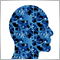69

Hello,

coming from MT4 I'm trying to implement some code in mql5.

A) an additional buffer is to be added to an already existing indicator with 3 buffers, to transmit a (buy, sell) condition to an EA.

Now in MT4 it works, when changing   #property indicator_buffers from 3 to 4, then adding to

SetIndexBuffer(0,Abuffer,INDICATOR_DATA);SetIndexBuffer(1,BBufffer,INDICATOR_DATA);  SetIndexBuffer(2,CBuffer,INDICATOR_DATA);

SetIndexBuffer(3,NewBuffer,INDICATOR_DATA); // double NewBuffer[] is already defined, btw

In this Indicator -   for the BuyCondition -  the NewBuffer is simply set to 1  ---- SellCondition set to -1

When addressing this indicator in an EA the "old" buffer-values(0,1,2) show up correctly using the Comment function.

When using CopyBuffer(HandleBuffer,0,0,2,BufferToImport);
ArraySetAsSeries(BufferToImport,true); etc. but in the case of CopyBuffer(HandleBuffer,3,0,2,BufferToImport) there appears just a very large number (looks like kinda overflow)

B) Does anyone know how to limit the number of bars, which are shown in the window and above all which are used to calculate in an indicator?

I though it would work by replacing  at the beginning    int OnCalculate(const int rates_total, .... by a constant number

int OnCalculate(const int 100,...for example but it doesn't work.

For any ideas thanks in advance

Mike6156

A).  The very large number is EMPTY_VALUEI suppose you will assign in each bar the value = 1 if there is a buy signal, the value = -1 if there is a sell signal and you will not assign any value if there is no signal of buy or sell.

In bars where there is no signal, you can assign the value 0 if you want, or you can also use the function:

PlotIndexSetDouble(3, PLOT_EMPTY_VALUE, 0.0);

B).  You should use:

PlotIndexSetInteger(3, PLOT_DRAW_BEGIN, begin);

Where begin is an integer = Number of initial (older) bars without drawing.

Ex: If you want to only show the most recent 100 bars:  begin = rates_total - 100

Regards.69

Many thanks for your excellent and fast support, Jose!

The topic under B) works perfectly

Re A) I set, just to test it, in the OnCalculate-part    PlotIndexSetDouble(3, PLOT_EMPTY_VALUE, 1);

the value 1 would be a buy-signal generated by the indicator.  A value of -1 would be a sell signal, btw.

Now, when using a code in the EA like CopyBuffer(Handle,0,0,2,Buffer); ArraySetAsSeries(Buffer,true); Comment(Buffer); the values of the original buffer0 are displayed correctly. But when I set it to receive the "signalbuffer values" CopyBuffer(Handle,3,0,2,Buffer) I only get 0.0 instead of 1

Maybe you could help me out solve this final step?